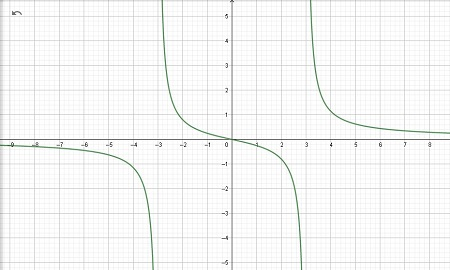# Explain how to graph rational expressions that have vertical and horizontal asymptotes.

## Question:

Explain how to graph rational expressions that have vertical and horizontal asymptotes.

## Graph of Rational Function

A rational function is any function that can be defined by a rational fraction, i.e. an algebraic fraction such that both the numerator and the denominator are polynomials. The coefficients of the polynomials need not be rational numbers; they may be taken in any field K.

If you are given a rational function you should know that it should have a vertical and horizontal asymptote.

A Vertical asymptotes are vertical lines which correspond to the zeroes of the denominator of a rational function.

To find the vertical asymptote we need to find the zeros of the denominator.

Example:

$$f(x)=\frac{2x}{x^{2}-9}$$

In this case,

we equate the denominator to zero,

$$x^{2}-9=0$$

Adding both sides by 9 we get,

$$x^{2}=9$$

Finding the square root of both sides we get,

$$\sqrt{x^{2}}=\sqrt{9}$$

So,

$$x=\pm 3$$

Therefore our vertical asymptotes are at x = -3 and x = 3.

On the other hand,

A horizontal asymptote is a y-value on a graph in which a function approaches but does not actually reach.

In order to find the horizontal asymptote, we need to compare the highest degree of the numerator and denominator.

Example:

$$f(x)=\frac{2x}{x^{2}-9}$$

we let

{eq}a {/eq} as the highest degree of the numerator.

{eq}b {/eq} as the highest degree of the denominator.

In this case, a = 1 and b = 2.

Note:

If {eq}a<b {/eq} then horizontal asymptote is the x-axis or at y=0.

If {eq}a=b {/eq} then horizontal asymptote is {eq}y=\frac{a}{b} {/eq}.

If {eq}a=b {/eq} then there is no horizontal asymptote.

Your last step is to plot the intercepts (if there are).

Example:

Graph {eq}f(x)=\frac{2x}{x^{2}-9} {/eq}vertical asymptote is at x=-3 and x=3

horizontal asymptote 1<2 so the horizontal asymptote is at y=0